# Hamster cage

Ryan keeps his hamster cage on his dresser. The area of the top of Ryan's dresser is 1 2\3 as large as the area of the bottom of his hamster cage. The area of the dresser top is 960 square inches. How many square inches of his dresser top are not covered by the hamster cage?

Correct result:

x =  640 sq inch

#### Solution: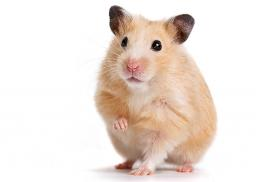We would be pleased if you find an error in the word problem, spelling mistakes, or inaccuracies and send it to us. Thank you!Tips to related online calculators
Need help calculate sum, simplify or multiply fractions? Try our fraction calculator.

#### You need to know the following knowledge to solve this word math problem:

We encourage you to watch this tutorial video on this math problem:

## Next similar math problems:

• PitPit has shape of a truncated pyramid with rectangular bases and is 0.8 m deep. The length and width of the pit is the top 3 × 1.5 m bottom 1 m × 0.5 m. To paint one square meter of pit we use 0.6 l of green colour. How many liters of paint is needed when
• GardenArea of a square garden is 6/4 of triangle garden with sides 56 m, 35 m, and 35 m. How many meters of fencing need to fence a square garden?
• Similarity n-gon9-gones ABCDEFGHI and A'B'C'D'E'F'G'H'I' are similar. The area of 9-gon ABCDEFGHI is S1=190 dm2 and the diagonal length GD is 32 dm. Calculate area of the 9-gon A'B'C'D'E'F'G'H'I' if G'D' = 13 dm.
• Three shapes1/5 of a circle is shaded. The ratio of area if square to the sum of area of rectangle and that of the circle is 1:2. 60% of the square is shaded and 1/3 of the rectangle is shaded. What is the ratio of the area of circle to that of the rectangle?
• Garden exchangeThe garden has the shape of a rectangular trapezoid, the bases of which have dimensions of 60 m and 30 m and a vertical arm of 40 m. The owner exchanged this garden for a parallelogram, the area of which is 7/9 of the area of a trapezoidal garden. What is
• GardensThe area of the square garden is 3/4 of the area of the triangular garden with sides of 80 m, 50 m, 50 m. How many meters of the fence do we need to fence a square garden?
• ConeCircular cone of height 15 cm and volume 5699 cm3 is at one-third of the height (measured from the bottom) cut by a plane parallel to the base. Calculate the radius and circumference of the circular cut.
• Gardens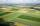I have a garden in the shape of a square with a side length of 0.02 kilometers. Neighbor has a large garden as well and one side of the garden we share. I'd bought from a neighbor garden that my area of my garden increased to 5 ares. a/ By how many acres
• Roof 8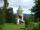How many liters of air are under the roof of tower which has the shape of a regular six-sided pyramid with a 3,6-meter-long bottom edge and a 2,5-meter height? Calculate the supporting columns occupy about 7% of the volume under the roof.
• PaintingTo paint the pool with dimensions: 2 meters depth, 3m x 4m we bought paint to 50 meters square. How many "paint" will be a waste?
• Picture frames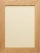Destiny has to make 24 picture frames. Each frame uses 16 3/8 wood trim. 2/3 were completed on the first day. How many inches of wood trim is needed to complete the remaining frames on the second day?
• Largest squaresHow many of the largest square sheets did the plumber cut the honeycomb from 16 dm and 96 dm?
• PresentGift box has rectangular shape with dimensions of 8×8×3 cm. Miloslav wants to cover with square paper with sides of 18 cm. How much paper left him?
• Pyramid roof2/4 of area of ​​the roof shaped regular tetrahedral pyramid with base edge 10 m and height of 4 m is already covered with roofing. How many square meters still needs to be covered?
• PizzaPizza with a diameter 50 cm have weight 559 g. What diameter will have a pizza weighing 855 g if it is make from the same cloth (same thickness....) and same decorated?
• GardenThe rectangular garden has dimensions of 27 m and 30 m. Peter and Katka split it in a ratio of 4:5. How many square meters did Katkin measure part of the garden?
• Cardboard box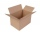Peter had square cardboard. The length of the pages was an integer in decimetres. He cut four squares with a side of 3 dm from the corners and made a box out of it, which fit exactly 108 cubes with an edge 1 dm long. Julia cut four squares with a side of